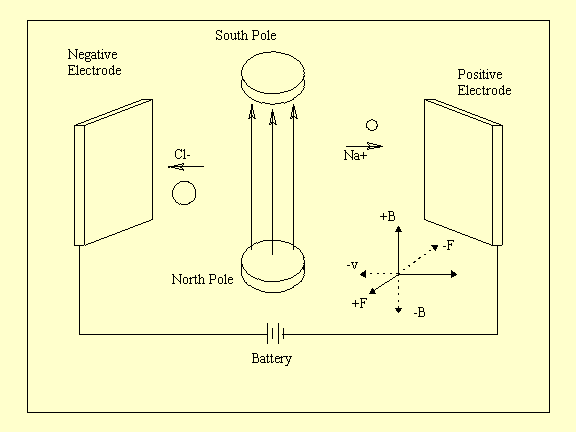The basic structure of the MHD mechanism is schematically represented in Figure 1. The two electrodes and two magnets are placed in a salt water solution (preferably saturated) so that the magnetic field is perpendicular to the current flowing through the water. The ions in the water will be attracted to their respective electrodes (opposite charges attract) creating a situation where the Lorentz force law can be applied.This schematic diagram can be used to find the direction of the force on the charged sodium and chlorine ions. To do this you can use the right hand rule directly, however remember that the right hand rule described here only applies to POSITIVELY charged particles. To fully understand how to apply the right hand rule to negatively charged particles you need to go into the concept of VECTOR CROSS PRODUCTS which is beyond the scope of this presentation ie: not covered in grade 12 physics.

A better way to figure out the direction of the force is to construct a set of axes, as shown in fig.1, and use the scalar equation of the Lorentz force law

To construct the axis you need the right hand rule. The positive velocity direction is in the direction of conventional current ie: the direction of the positive charge flow (+ to -). All the other positive axes follows from the right hand rule (Thumb in + v direction ...).

Now using F = QvB you ignore the actual numbers of Q, v, B (since they would be difficult to measure) and work with the SIGNS of the numbers. In the cases of v and B, the signs correspond to the respective DIRECTIONS of v and B according to the axes you just set up. For Q the sign corresponds to whether the particle is positively charged or negatively charged (in this case Na+ or Cl-). Thus the sign of the resultant force is just found by multiplying all the signs together, according to F = QvB, and the direction is found from the axes you constructed.

For example refer to fig.1 and take Cl- :

• The sign of Q is -
• The sign of v is -
• The sign of B is +
• Therefore two negatives and a positive all multiplied together gives a positive and so the force on the chlorine ion is in the positive F direction which is out of the page. The same can be done for the sodium ion and the resultant force is in the same direction.